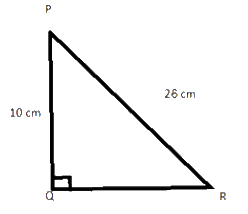# Ex.6.5 Q1 The Triangle and Its Properties - NCERT Maths Class 7

## Question

$$PQR$$ is a triangle right angled at $$P.$$ If $$PQ$$ $$=\rm{}\,10\, cm$$ and $$PR$$ $$= \rm{}\,24\, cm,$$ find $$QR.$$

Video Solution
Triangle & Its Properties
Ex 6.5 | Question 1

## Text Solution

What is Known?

$$PQR$$ is a triangle right angled at $$P$$ and the length of two sides $$PQ$$ $$=\rm{}\,10\,cm$$ and $$PR$$ $$= \rm{}\,24\, cm.$$

What is unknown?

Length of one side of the triangle.

Reasoning:

This question is straight forward, as it is given in the question that $$PQR$$ is a right- angled triangle and it is right angled at $$P.$$ So, we can apply Pythagoras theorem here, if it is right angled at $$P$$ then the side opposite to $$P$$ will be the hypotenuse of the triangle i.e.$$QR$$ and the other side is given $$PQ$$ $$= \rm{}\,10\,cm$$ and $$PR=24$$. Now, by applying Pythagoras theorem i.e. in a right-angled triangle, the square of hypotenuse is equal to the sum of square of other two sides, we can find $$QR.$$ For better visual understanding draw a right-angled triangle which is right angled at $$Q$$ and consider the side opposite to it $$PR$$ as hypotenuseSteps:

Given, $$PQ$$ $$= \rm{}\,10\,cm,$$ $$PR$$ $$= \rm{}\,24\,cm$$ and $$QR =?$$

By applying Pythagoras theorem in triangle $$PQR,$$ we get

\begin{align} \left( {{\rm{Hypotenuse}}} \right)^2 & \!=\! \left[ \begin{array}{l} \left( {{\rm{Perpendicular}}} \right)^2 \!+\! \\ \left( {{\rm{Base}}} \right)^2 \\ \end{array} \right] \\ QR^2 &\!=\! \left( {{\rm{PQ}}} \right)^2 \!+\! \left( {{\rm{PR}}} \right)^2 \\ QR^2 &\!=\! \left( {{\rm{10}}} \right)^2 \!+\! \left( {{\rm{24}}} \right)^2 \\ QR^2 &\!=\! 100 \!+\! 576 \\ QR^2 &\!=\! 676 \\ QR &\!=\! 26\;{\rm{cm}} \\ \end{align}

Thus, $$QR$$ is equal to $$26$$ cm

Useful Tip:

Whenever you encounter problems of this kind, it is best to think of the Pythagoras theorem for right angled triangle.

Learn from the best math teachers and top your exams

• Live one on one classroom and doubt clearing
• Practice worksheets in and after class for conceptual clarity
• Personalized curriculum to keep up with school